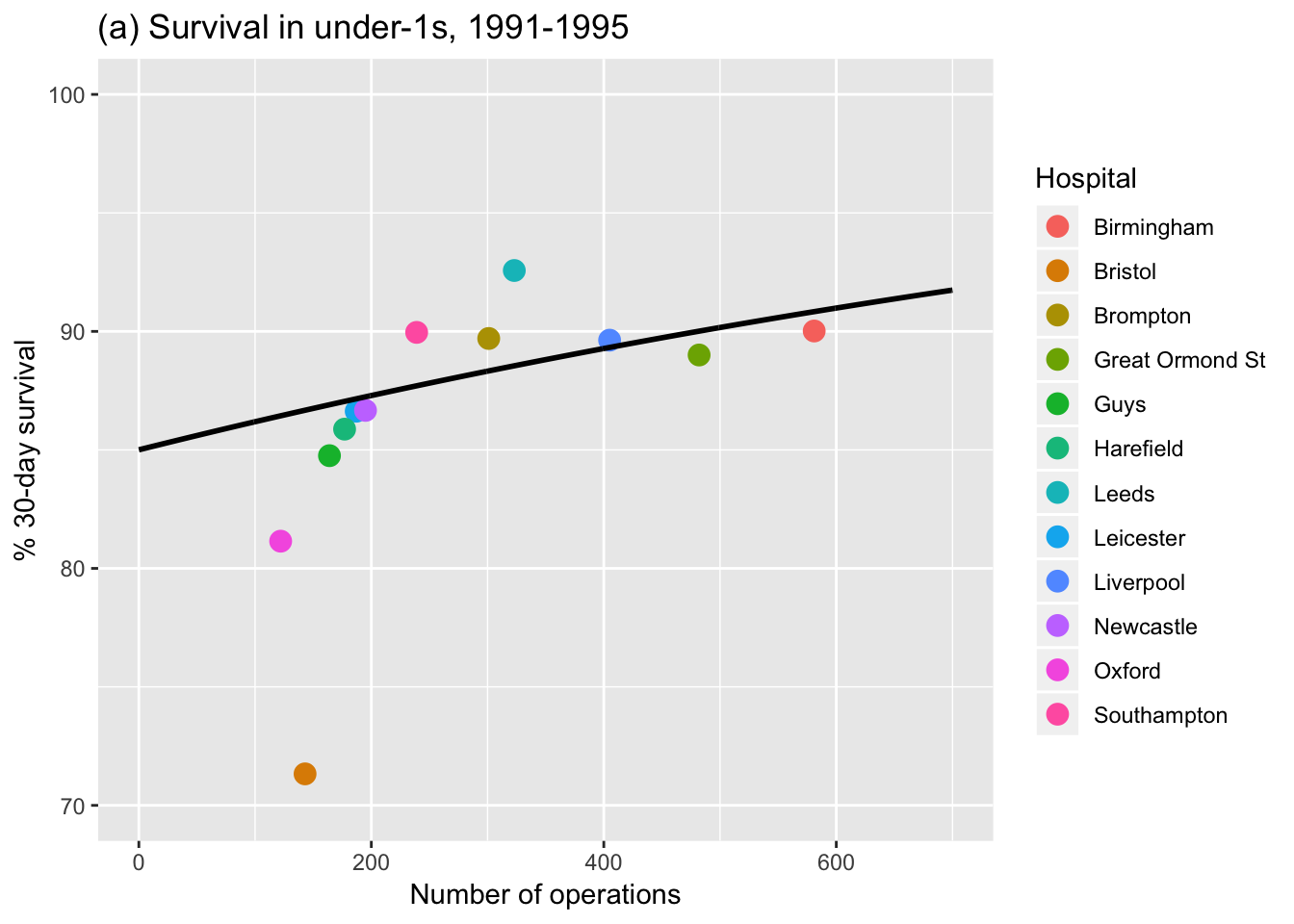Data from 1991-1995 are contained in 02-5-child-heart-surgery-1991-x.csv, and taken from D.J. Spiegelhalter et al., Commissioned Analysis of Surgical Performance Using Routine Data: Lessons from the Bristol Inquiry.

library(ggplot2)

attach(child.1991)
# leave first row (Bristol) out of the fit
fit=glm(Survivors/Operations ~ Operations, weight=Operations, family="binomial",data=child.1991[-1,])
summary(fit)
##
## Call:
## glm(formula = Survivors/Operations ~ Operations, family = "binomial",
##     data = child.1991[-1, ], weights = Operations)
##
## Deviance Residuals:
##     Min       1Q   Median       3Q      Max
## -1.6266  -0.6994  -0.2385   0.4765   2.4074
##
## Coefficients:
##              Estimate Std. Error z value Pr(>|z|)
## (Intercept) 1.7348545  0.1410843  12.297   <2e-16 ***
## Operations  0.0009615  0.0003807   2.526   0.0115 *
## ---
## Signif. codes:  0 '***' 0.001 '**' 0.01 '*' 0.05 '.' 0.1 ' ' 1
##
## (Dispersion parameter for binomial family taken to be 1)
##
##     Null deviance: 18.623  on 10  degrees of freedom
## Residual deviance: 12.169  on  9  degrees of freedom
## AIC: 72.622
##
## Number of Fisher Scoring iterations: 4
predictions=100*predict(fit, data.frame(Operations=0:700), type="response")  # predictions for extreme cases

pred.frame = data.frame(Extremes=0:700,predictions ) # data frame for predictions

p <- ggplot()
p <- p + geom_point(child.1991, mapping=aes(x=Operations, y=100*Survivors/Operations, col=Hospital), size=3.5) # defines scatter-type plot, plot axis data fields and colour legend data field
p <- p + expand_limits(x = c(0,700),y=c(70,100))
p <- p +  labs(x="Number of operations", y = "% 30-day survival", title="(a) Survival in under-1s, 1991-1995") # Adds axes and title
p <- p + geom_line(dat=pred.frame, aes(x=Extremes,y=predictions), size=1) # add previously fitted logstic regression line

pFigure 5.2 Fitted logistic regression model for child heart surgery data for under-1s in UK hospitals between 1991 and 1995. Hospitals treating more patients have better survival. The line is part of a curve that will never reach 100%, and is fitted ignoring the outlying data-point representing Bristol.